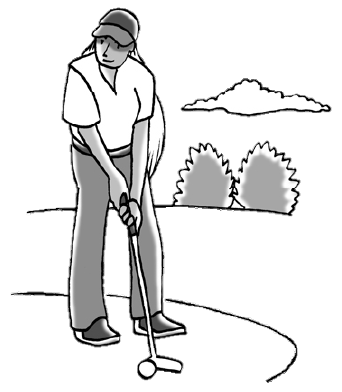### Home > INT3 > Chapter 6 > Lesson 6.3.1 > Problem6-80

6-80.

MINIATURE GOLFOlivia loves playing putt-putt golf. In putt-putt golf a person does not swing at a golf ball, but rather only putts the golf ball (taps the ball with a club so that it rolls into a hole). Olivia experiments with her new club to determine whether or not she plays better with it. Each time before she putts a golf ball, Olivia flips a coin to determine whether she will use her new club or an old one. She experiments on $80$ putts. With the new club, Olivia makes $25$% of the $40$ putts while with the old one, she makes only $15$% of the $40$ putts. Using the steps below, help Olivia decide whether or not her new golf club is really better, or if this difference can be explained by sample-to-sample variability.

1. What is the difference in the proportions (new club minus old club)? Express your answer as a decimal.

$25\%−15\%$

2. Olivia needs a computer simulation to determine the sample-to-sample variability:

1. Out of the $80$ putts, how many go into the hole?

$(25\% \text{ of } 40) + (15\% \text{ of } 40)$

2. A model of this situation needs to represent $80$ putts. What will the numbers $1$ to $16$ represent? What will the numbers $17$ to $80$ represent?

$16$ out of Olivia's $80$ putts went in the hole.

3. Conduct one simulation of $80$ putts. What proportion of the putts in your simulation go into the hole? What proportion do not go into the hole? What is the difference in the proportion (proportion that goes into the hole minus proportion that does not go in)?

Using a graphing calculator, randlnt$(0,1, 80)$. Let $0 =$ a miss.

Olivia runs the simulation $50$ times, and for each simulation, she calculates the difference in the proportion of putts that go into the hole and those that do not go into the hole. From her results, she predicts the true difference in proportion of all her putts is $0.10\pm0.146$.

3. What does a difference of zero mean in the context of this problem? Is a difference of zero a plausible result considering your margin of error?

The margin of error is from $(0.10 − 0.146)$ to $(0.10 + 0.146)$.
Is $0$ within the margin of error?

4. Are you convinced that there is a true difference between the new club and the old club?